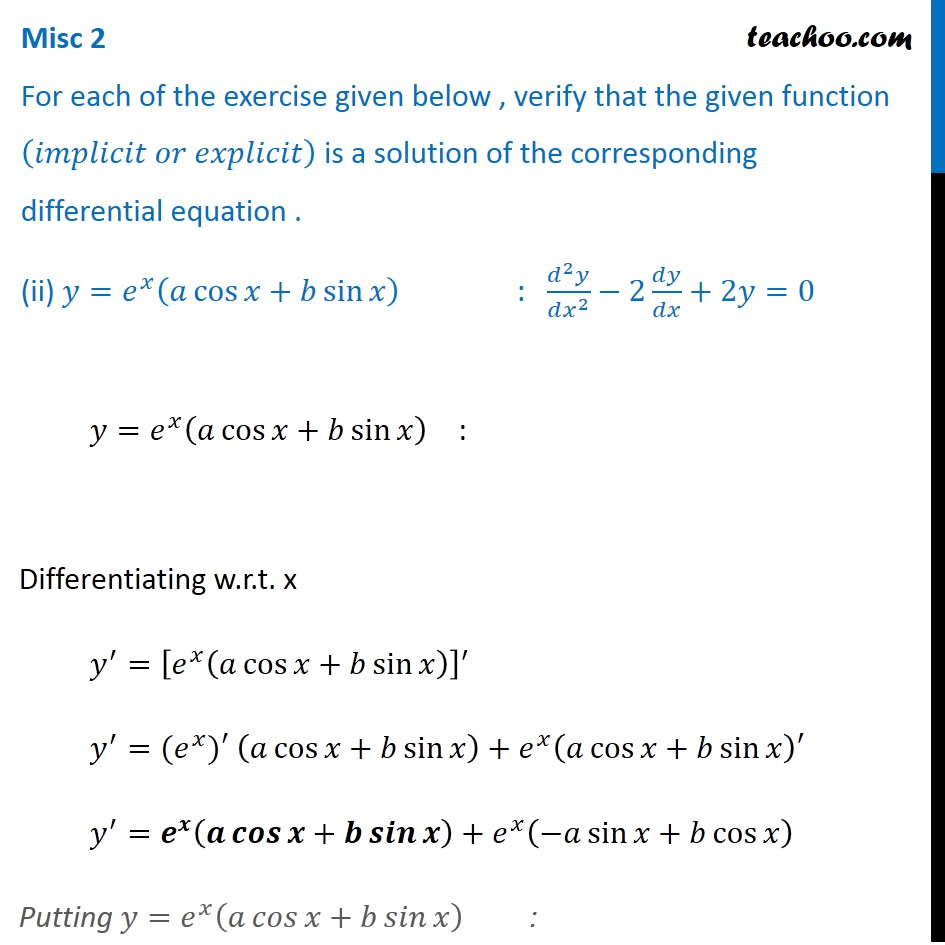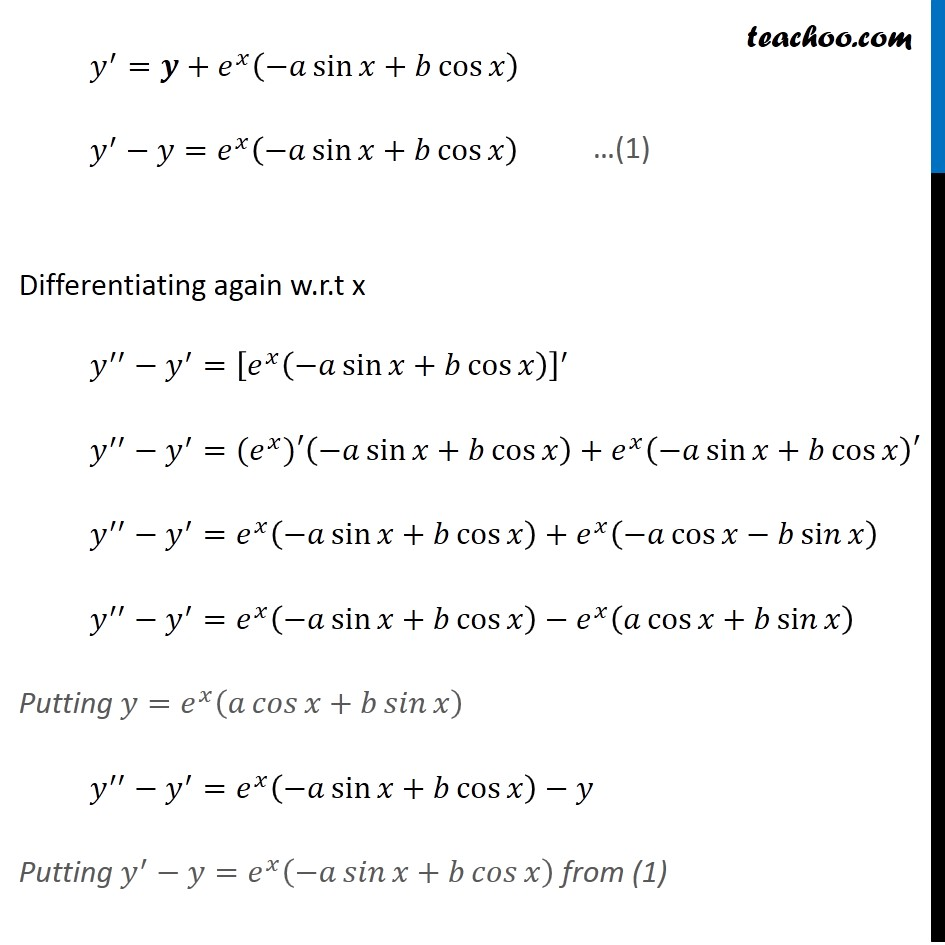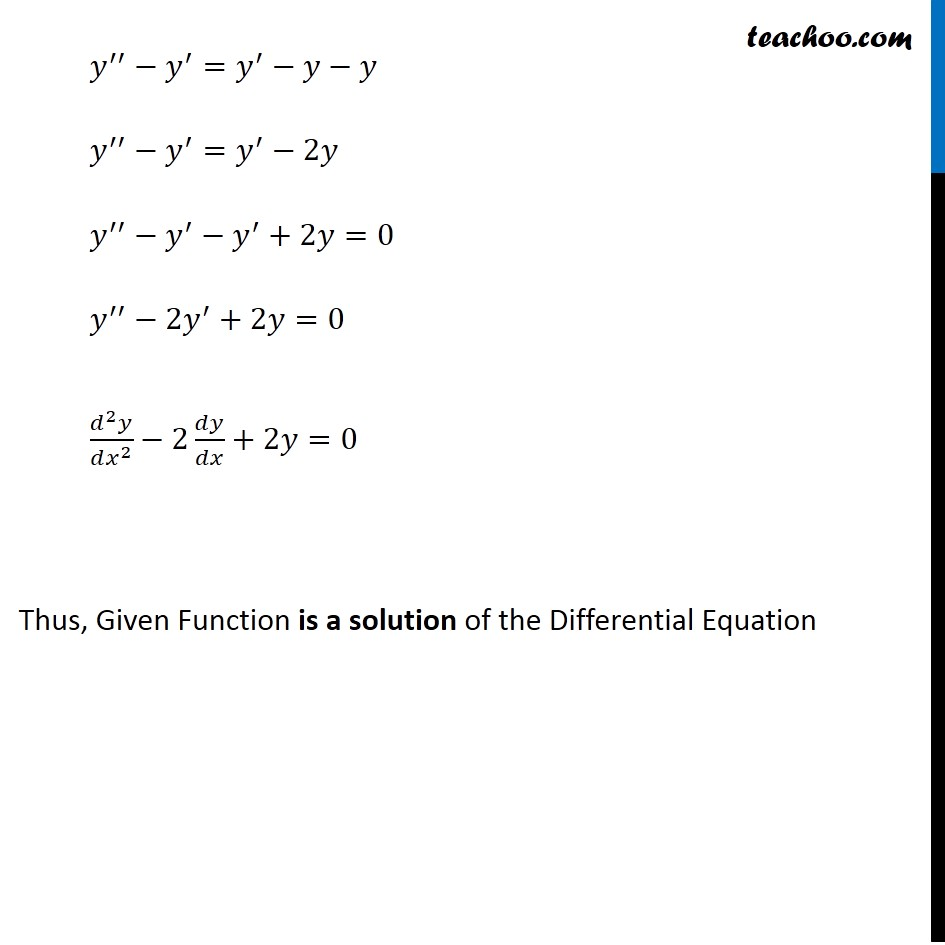Miscellaneous

Chapter 9 Class 12 Differential Equations
Serial order wiseGet live Maths 1-on-1 Classs - Class 6 to 12

### Transcript

Misc 2 For each of the exercise given below , verify that the given function (𝑖𝑚𝑝𝑙𝑖𝑐𝑖𝑡 𝑜𝑟 𝑒𝑥𝑝𝑙𝑖𝑐𝑖𝑡) is a solution of the corresponding differential equation . (ii) 𝑦=𝑒^𝑥 (𝑎 cos⁡〖𝑥+𝑏 sin⁡𝑥 〗 ) : (𝑑^2 𝑦)/(𝑑𝑥^2 )−2 𝑑𝑦/𝑑𝑥+2𝑦=0 𝑦=𝑒^𝑥 (𝑎 cos⁡〖𝑥+𝑏 sin⁡𝑥 〗 ) : Differentiating w.r.t. x 𝑦^′=[𝑒^𝑥 (𝑎 cos⁡〖𝑥+𝑏 sin⁡𝑥 〗 )]^′ 𝑦^′=〖(𝑒〗^𝑥)′ (𝑎 cos⁡〖𝑥+𝑏 sin⁡𝑥 〗 )+𝑒^𝑥 (𝑎 cos⁡〖𝑥+𝑏 sin⁡𝑥 〗 )′ 𝑦^′=𝒆^𝒙 (𝒂 𝒄𝒐𝒔⁡〖𝒙+𝒃 𝒔𝒊𝒏⁡𝒙 〗 )+𝑒^𝑥 (−𝑎 sin⁡〖𝑥+𝑏 cos⁡𝑥 〗 ) Putting 𝑦=𝑒^𝑥 (𝑎 𝑐𝑜𝑠⁡〖𝑥+𝑏 𝑠𝑖𝑛⁡𝑥 〗 ) : 𝑦^′=𝒚+𝑒^𝑥 (−𝑎 sin⁡〖𝑥+𝑏 cos⁡𝑥 〗 ) 𝑦^′−𝑦=𝑒^𝑥 (−𝑎 sin⁡〖𝑥+𝑏 cos⁡𝑥 〗 ) Differentiating again w.r.t x 𝑦^′′−𝑦^′=[𝑒^𝑥 (−𝑎 sin⁡〖𝑥+𝑏 cos⁡𝑥 〗 )]^′ 𝑦^′′−𝑦^′=〖(𝑒〗^𝑥)′(−𝑎 sin⁡〖𝑥+𝑏 cos⁡𝑥 〗 )+𝑒^𝑥 (−𝑎 sin⁡〖𝑥+𝑏 cos⁡𝑥 〗 )′ 𝑦^′′−𝑦^′=𝑒^𝑥 (−𝑎 sin⁡〖𝑥+𝑏 cos⁡𝑥 〗 )+𝑒^𝑥 (−𝑎 cos⁡〖𝑥−𝑏 si𝑛⁡𝑥 〗 ) 𝑦^′′−𝑦^′=𝑒^𝑥 (−𝑎 sin⁡〖𝑥+𝑏 cos⁡𝑥 〗 )−𝑒^𝑥 (𝑎 cos⁡〖𝑥+𝑏 si𝑛⁡𝑥 〗 ) Putting 𝑦=𝑒^𝑥 (𝑎 𝑐𝑜𝑠⁡〖𝑥+𝑏 𝑠𝑖𝑛⁡𝑥 〗 ) 𝑦^′′−𝑦^′=𝑒^𝑥 (−𝑎 sin⁡〖𝑥+𝑏 cos⁡𝑥 〗 )−𝑦 Putting 𝑦^′−𝑦=𝑒^𝑥 (−𝑎 𝑠𝑖𝑛⁡〖𝑥+𝑏 𝑐𝑜𝑠⁡𝑥 〗 ) from (1) …(1) 𝑦^′′−𝑦^′=𝑦^′−𝑦−𝑦 𝑦^′′−𝑦^′=𝑦^′−2𝑦 𝑦^′′−𝑦^′−𝑦^′+2𝑦=0 𝑦^′′−2𝑦^′+2𝑦=0 (𝑑^2 𝑦)/(𝑑𝑥^2 )−2 𝑑𝑦/𝑑𝑥+2𝑦=0 Thus, Given Function is a solution of the Differential Equation